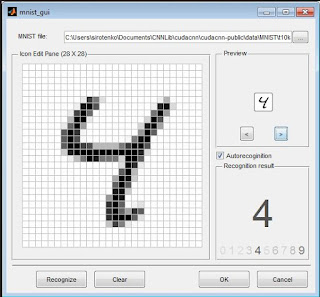# What is the Difference between Classification and Regression

Today we will understand the difference between classification and regression. It would be a very short lesson but at the end it would be very clear what the differences are.
Remember that both machine learning concepts are the two aspects of supervised learning, where you have a training data set.

Just as you know, Supervised learning algorithms is divided into two broad  categories: classification and regression.

Classification
In classification, the objective is to assign each of the input vector to one of a given number of discrete categories. An algorithm that implements classification is known as a classifier.Figure 1: Digit recognition of the digit 4

When there are only two classes, then the technique is known as binary classification and the algorithm is called a binary classifier.
A typical example of classification is the image recognition problem. The input is a handwritten image made up of a grid of pixels, say 64 x 64. And the aim is to assign each image to one of 10 classes, 1 to 9. This is illustrated in Figure 1.

• Application areas of classification includes:
• Handwriting recognition
• Computer vision
• Voice recognition
• Internet Search Engines
• Natural language processing

Regression
In the case of regression the objective is to find the a relationship among the input variables. Regression analysis helps in understanding how the dependent variable changes with respect to the independent variables.Figure 2: Linear Regression in Classification

In case of linear regression, the objective is to fit a straight line through the data points to obtain the best fit.

Techniques for Regression includes:

• Simple Linear Regression